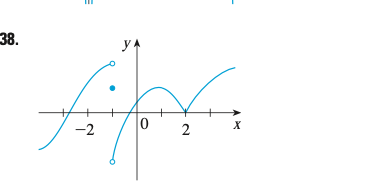💬 👋 We’re always here. Join our Discord to connect with other students 24/7, any time, night or day.Join Here!

DM

Diyor M.

Calculus 2 / BC

1 week, 5 days ago

# The graph of f is given. State, with reasons, the numbers at which f is not differentiable.Chandigarh University

The graph of f is given. State, with reasons, the numbers at which f is not differentiable.

### Discussion

You must be signed in to discuss.

### Video Transcript

in this there is a graph of F which has been given and I have to Straight with regions. The number at 58 F. is not differentiable, first of all. For differential ability, there should be a number. That is the first condition. There should be a point where we can draw the tangent and second age. The craft should be differential. Girls should be continuous at that point and there should not be any corners like this one and there should not be any discontinuity at the craft. Well, we intend to find The differentiation. Okay, so we can easily see that this is .1. So very discontinuity at this point X equal to one and there is a corner like age 0.2. So it is not differentiate. It cannot be differentiated at tax equal to one and X equal to to this minus, That's equal to -1 and X equal to to This graph is not differentiable at. There's no points available points here where we can draw attendant and there are many more. There are in fact many tensions can be drawn at X equal to two. Thank you.Chandigarh University
##### Top Calculus 2 / BC Educators##### Catherine R.

Missouri State University##### Samuel H.

University of Nottingham